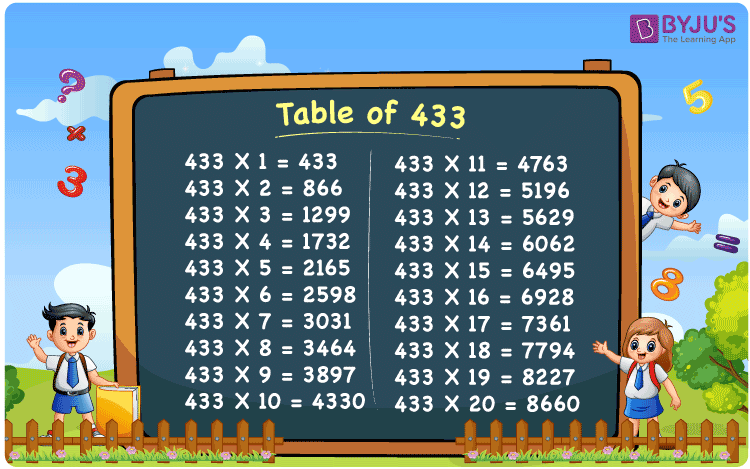Checkout JEE MAINS 2022 Question Paper Analysis : Checkout JEE MAINS 2022 Question Paper Analysis :

# Table of 433

The Table of 433 up to 20 times is available here for free. Students can also download the table of 433 and use it to solve mathematical problems quickly. 433 times table will represent here the multiples of the original number. 433 times any number is equivalent to repeated addition of it, respectively. For example, 433 x 3 = 433 + 433 + 433 = 1299. Learn more about Multiplication tables at BYJU’S.

## Table of 433 Chart## What is 433 Times Table?

The table below shows the repeated addition of the number 433 to get the required multiple.

 433×1 = 433 433 433×2 = 866 433 + 433 = 866 433×3 = 1299 433 + 433 + 433 = 1299 433×4 = 1732 433 + 433 + 433 + 433 = 1732 433×5 = 2165 433 + 433 + 433 + 433 + 433 = 2165 433×6 = 2598 433 + 433 + 433 + 433 + 433 + 433 = 2598 433×7 = 3031 433 + 433 + 433 + 433 + 433 + 433 + 433 = 3031 433×8 = 3464 433 + 433 + 433 + 433 + 433 + 433 + 433 + 433 = 3464 433×9 = 3897 433 + 433 + 433 + 433 + 433 + 433 + 433 + 433 + 433 = 3897 433×10 = 4330 433 + 433 + 433 + 433 + 433 + 433 + 433 + 433 + 433 + 433 = 4330

## Multiplication Table of 433

Here is the table of 433 up to 20 times below.

 433 × 1 = 433 433 × 2 = 866 433 × 3 = 1299 433 × 4 = 1732 433 × 5 = 2165 433 × 6 = 2598 433 × 7 = 3031 433 × 8 = 3464 433 × 9 = 3897 433 × 10 = 4330 433 × 11 = 4763 433 × 12 = 5196 433 × 13 = 5629 433 × 14 = 6062 433 × 15 = 6495 433 × 16 = 6928 433 × 17 = 7361 433 × 18 = 7794 433 × 19 = 8227 433 × 20 = 8660

## Solved Example on Table of 433

Find: 433 x 5 + 2000

Solution: Given,

433 x 5 + 2000

From the table of 433, we have;

433 x 5 = 2165

Therefore,

2165 – 2000 = 165

## Frequently Asked Questions on Table of 433

### What is table of 433?

In Mathematics, the table of 433 includes all the multiples of 433 when multiplied by a set of positive integers.

### What is the value of 433 x 8?

The value of 433 x 8 is equal to 3464.

### What is the 12th multiple of 433?

The 12th multiple of 433 is 5196.